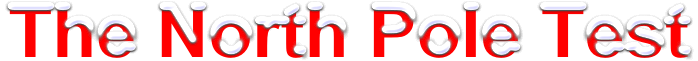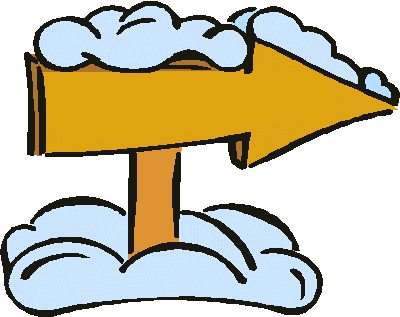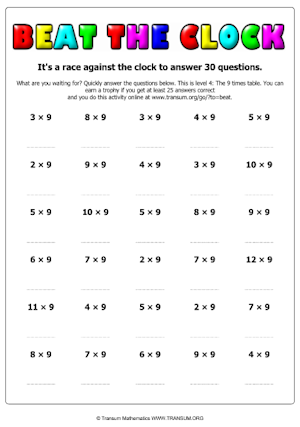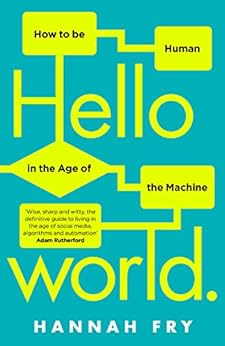#It is very cold at the North Pole, so cold that your brain could stop working.

Show that your brain is working well right now by writing out the 112 times table.

How far can you get in five minutes?North Pole
112 miles

Try this activity again when you are feeling cold. Can you get as far in five minutes?## A Chilling Mathematics Lesson Starter Of The Day

Share

Topics: Starter | Tables | Xmas

You can find many more ChristMaths activities at:

Transum.org/ChristMaths/• Primary 6 & Mrs Thom, Netherlee PS
•
• In five minutes, our primary 6 class managed to get the first 16 stations correct. We felt that it was tricky but a lot of fun. Some were frustrated that if they got one answer wrong then every other answer was also wrong.

We really enjoyed today's challenge!
• Matthew, 3D, Craigslea State School
•
• I got up to the 29th station in 5 minutes.
It was fun!
• Katee Wheeler, ------
•
• This starter has some potential and is very helpful and interesting for developing Mathematical skills.
• Debbie, Guernsey
•
• Very absorbing activity! One of my Y5s managed up to 49 x 44 in 5 minutes.

[Transum: If are reading this comment and wondering why it refers to the 44 times table while you have been presented with another times table it is because a different number appears every time this page is loaded.]
• Transum,
•
• Yes, the cold can really affect your brain in a negative way. Perhaps a chilly day where you live won’t make a big difference but for really cold environments there have been studies which have found that mental changes (reflecting a cooling brain) are the first sign of hypothermia. The tell-tale signs include becoming withdrawn, slower movements, poor coordination, hallucinations, slurred speech, poor memory and eventually loss of consciousness.

How did you use this starter? Can you suggest how teachers could present or develop this resource? Do you have any comments? It is always useful to receive feedback and helps make this free resource even more useful for Maths teachers anywhere in the world.

If you don't have the time to provide feedback we'd really appreciate it if you could give this page a score! We are constantly improving and adding to these starters so it would be really helpful to know which ones are most useful. Simply click on a button below:

Excellent, I would like to see more like this
Good, achieved the results I required
Satisfactory
Didn't really capture the interest of the students
Not for me! I wouldn't use this type of activity.

This starter has scored a mean of 3.0 out of 5 based on 179 votes.

Previous Day | This starter is for 17 December | Next Day

1 x 112 = 112
2 x 112 = 224
3 x 112 = 336
4 x 112 = 448
5 x 112 = 560
6 x 112 = 672
7 x 112 = 784
8 x 112 = 896
9 x 112 = 1008
10 x 112 = 1120
11 x 112 = 1232
12 x 112 = 1344
13 x 112 = 1456
14 x 112 = 1568
15 x 112 = 1680
16 x 112 = 1792
17 x 112 = 1904
18 x 112 = 2016
19 x 112 = 2128
20 x 112 = 2240
21 x 112 = 2352
22 x 112 = 2464
23 x 112 = 2576
24 x 112 = 2688
25 x 112 = 2800
26 x 112 = 2912
27 x 112 = 3024
28 x 112 = 3136
29 x 112 = 3248
30 x 112 = 3360
31 x 112 = 3472
32 x 112 = 3584
33 x 112 = 3696
34 x 112 = 3808
35 x 112 = 3920
36 x 112 = 4032
37 x 112 = 4144
38 x 112 = 4256
39 x 112 = 4368
40 x 112 = 4480
41 x 112 = 4592
42 x 112 = 4704
43 x 112 = 4816
44 x 112 = 4928
45 x 112 = 5040
46 x 112 = 5152
47 x 112 = 5264
48 x 112 = 5376
49 x 112 = 5488
50 x 112 = 5600
51 x 112 = 5712
52 x 112 = 5824
53 x 112 = 5936
54 x 112 = 6048
55 x 112 = 6160
56 x 112 = 6272
57 x 112 = 6384
58 x 112 = 6496
59 x 112 = 6608
60 x 112 = 6720
61 x 112 = 6832
62 x 112 = 6944
63 x 112 = 7056
64 x 112 = 7168
65 x 112 = 7280
66 x 112 = 7392
67 x 112 = 7504
68 x 112 = 7616
69 x 112 = 7728
70 x 112 = 7840
71 x 112 = 7952
72 x 112 = 8064
73 x 112 = 8176
74 x 112 = 8288
75 x 112 = 8400
76 x 112 = 8512
77 x 112 = 8624
78 x 112 = 8736
79 x 112 = 8848
80 x 112 = 8960
81 x 112 = 9072
82 x 112 = 9184
83 x 112 = 9296
84 x 112 = 9408
85 x 112 = 9520
86 x 112 = 9632
87 x 112 = 9744
88 x 112 = 9856
89 x 112 = 9968
90 x 112 = 10080
91 x 112 = 10192
92 x 112 = 10304
93 x 112 = 10416
94 x 112 = 10528
95 x 112 = 10640
96 x 112 = 10752
97 x 112 = 10864
98 x 112 = 10976
99 x 112 = 11088
100 x 112 = 11200

Note to teacher: Doing this activity once with a class helps students develop strategies. It is only when they do this activity a second time that they will have the opportunity to practise those strategies. That is when the learning is consolidated. Click the button above to regenerate another version of this starter from random numbers.

There are a printable worksheets for the more common timestables.Your access to the majority of the Transum resources continues to be free but you can help support the continued growth of the website by doing your Amazon shopping using the links on this page. Below is an Amazon search box and some items chosen and recommended by Transum Mathematics to get you started.

## Hello World

You are buying a (driverless) car. One vehicle is programmed to save as many lives as possible in a collision. Another promises to prioritize the lives of its passengers. Which do you choose?

Welcome to the age of the algorithm, the story of a not-too-distant future where machines rule supreme, making important decisions – in healthcare, transport, finance, security, what we watch, where we go even who we send to prison. So how much should we rely on them? What kind of future do we want?

Hannah Fry takes us on a tour of the good, the bad and the downright ugly of the algorithms that surround us. In Hello World she lifts the lid on their inner workings, demonstrates their power, exposes their limitations, and examines whether they really are an improvement on the humans they are replacing. more...Teacher, do your students have access to computers?Do they have iPads or Laptops in Lessons? Whether your students each have a TabletPC, a Surface or a Mac, this activity lends itself to eLearning (Engaged Learning).Here a concise URL for a version of this page without the comments.

Transum.org/go/?Start=December17

Here is the URL which will take them to a game that will help them practise the 112 times table.

Transum.org/go/TD/?T=112

Here is the URL which will take them to the most popular multiplication tables app.

Transum.org/go/?to=tablesmasterFor All: